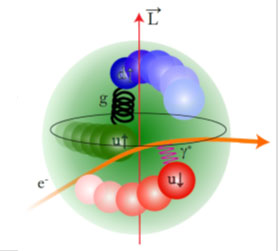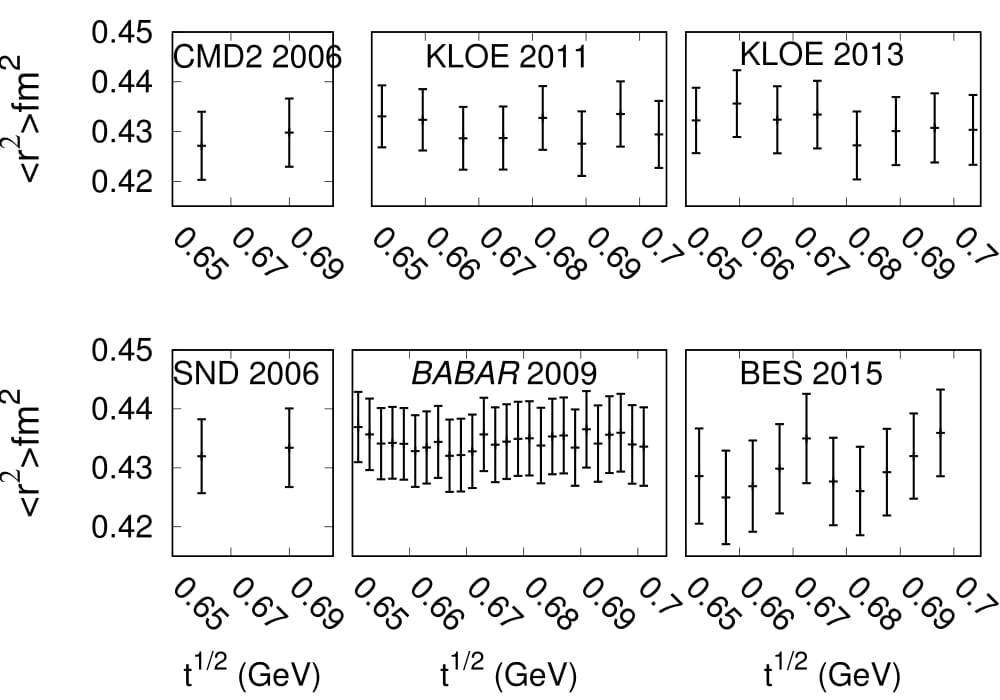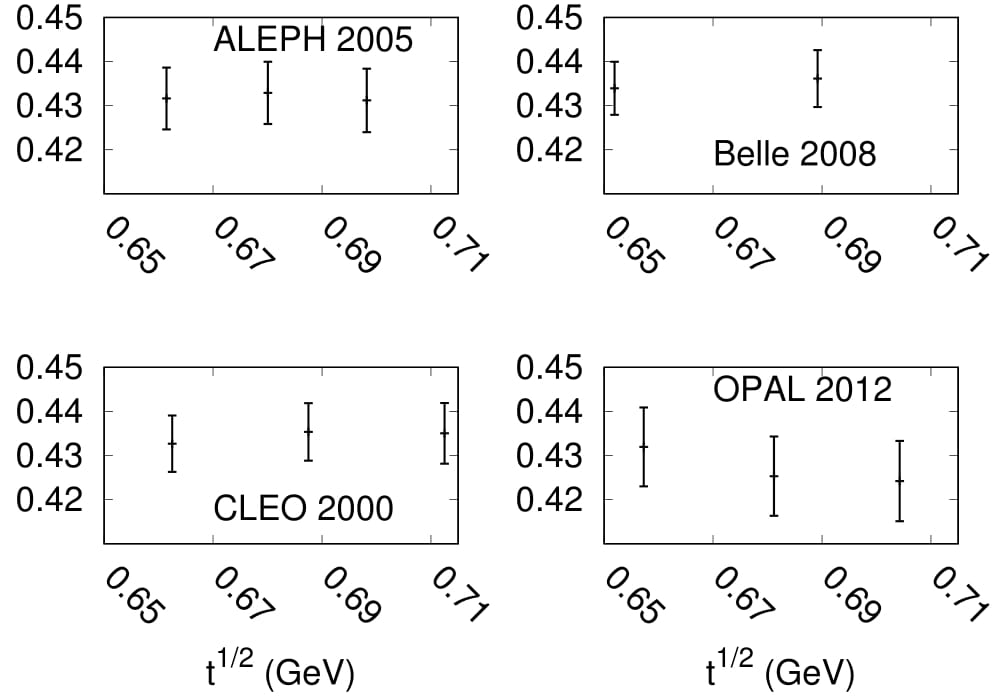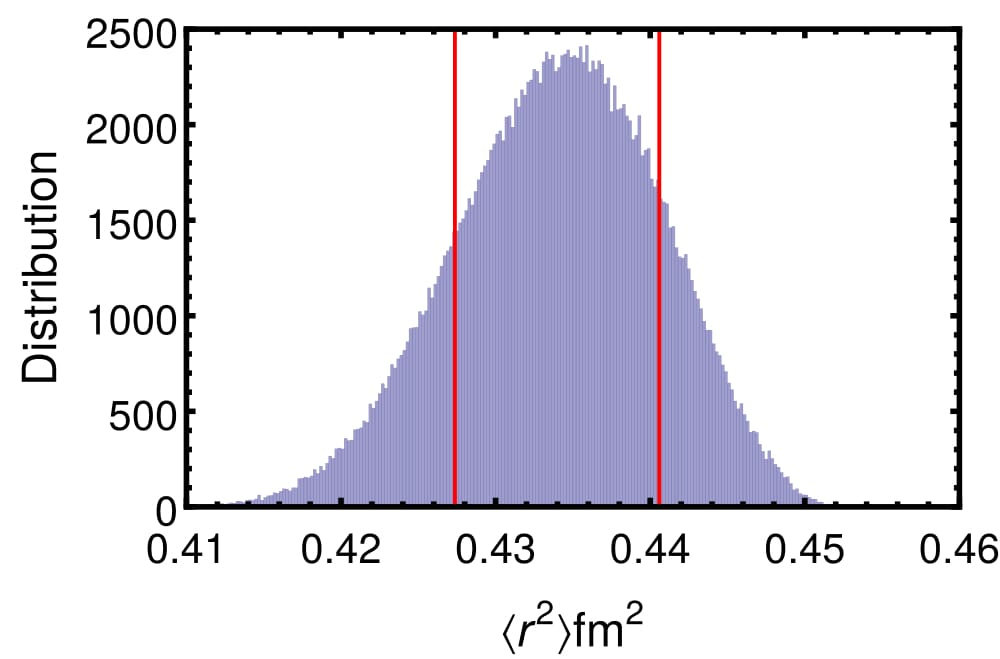# Precise determination of the charge radius of the pionFigure depicting structure of the proton. An analogous study is done here for the pionThe fundamental building blocks of matter are the elementary particles that can not be further broken into smaller constituents, for example an electron. There are a type of elementary particles, called the quarks that are electrically charged, are the building blocks of composite particles such as protons and neutrons that we know form the nucleus of an atom. Depending on how many quarks a composite particle is made of, it could have a net electric charge. Elementary particles are so small that their electric charge is considered concentrated in a point in space. However for composite particles, due to their finite size, the electric charge is smeared out over small region of space. The spatial extent of this charge distribution is known as the charge radius and is a measure of the size of the particle, where the square of the radius equals 6 times the derivative of the form factor with respect to the square of the momentum transfer at zero momentum transfer, where the form factor is the function that describes the structure of the pion.The charge radius of a pion which is made of two quarks, has been of interest for over half a century and is an important quantity of the theory of strong interaction, a theory that explains how quarks are bound together inside a pion or a proton. However it is extremely difficult to determine the charge radius of a pion as pions decay very fast into other particles (for example a charged pion decay within a billionth of a nanoseconds after its production).

One way to probe the charge radius is through electromagnetic interactions, such as pion production in electron-positron annihilation, scattering of pions from protons etc. Decays of tau-lepton, a heavier cousin of electron is also useful in this regard. High precision from these measurements can be used to extract the form factor at certain energies. In a recent theoretical work done at IISc (see references below),B. Ananthanarayan along with long term collaborators Irinel Caprini (Bucharest) and Diganta Das (Ahmedabad and Delhi) has used a mathematical framework that is based on the principle of quantum mechanics called unitarity and analyticity to correlate the pion charge radius with experimental data. Since uncertainties are inherent to all experimental data they have used a sophisticated statistical analysis technique and has extracted the pion charge radius using the mathematical framework to be 0.657 femtometer with an uncertainty of 0.003 femtometer. The uncertainty in their determination is about three times smaller compared to what is adopted by the Particle Data Group making it the most precise value of pion charge radius extracted to date.